Seventh Mississippi State - UAB Conference on Differential Equations and Computational Simulations. Electron. J. Diff. Eqns., Conference 17 (2009), pp. 81-94.

### A third-order m-point boundary-value problem of Dirichlet type involving a p-Laplacian type operator Chaitan P. Gupta

Abstract:
Let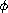, be an odd increasing homeomorphisms from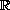ontosatisfying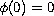, and let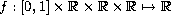be a function satisfying Caratheodory's conditions. Let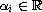,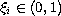,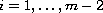,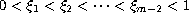be given. We are interested in the existence of solutions for the-point boundary-value problem: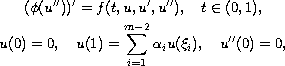in the resonance and non-resonance cases. We say that this problem is at \emph{resonance} if the associated problem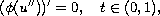with the above boundary conditions has a non-trivial solution. This is the case if and only if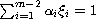. Our results use topological degree methods. In the non-resonance case; i.e., when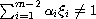we note that the sign of degree for the relevant operator depends on the sign of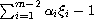.

Published April 15, 2009.
Math Subject Classifications: 34B10, 34B15, 34L30.
Key Words: m-point boundary value problems; p-Laplace type operator; non-resonance; resonance; topological degree.

Show me the PDF file (270 KB), TEX file, and other files for this article.Chaitan P. Gupta Department of Mathematics, 084 University of Nevada Reno, NV 89557, USA email: gupta@unr.edu